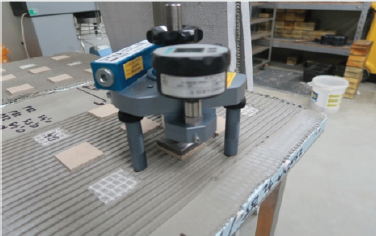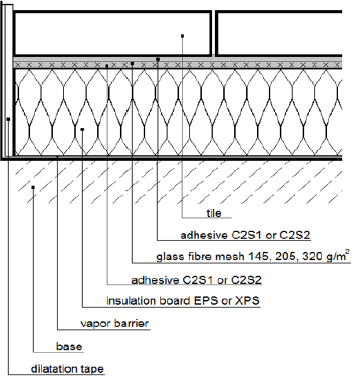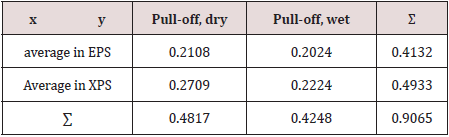Email Us: info@lupinepublishers.comCall Us: +1 (914) 407-6109   57 West 57th Street, 3rd floor, New York - NY 10019, USA

# Lupine Publishers Group

## Lupine Publishers

Submit Manuscript

ISSN: 2641-6921# Regression of The Tensile Strength of Deformable Adhesive From Thermal Insulation Density In The Lightweight Floor System Volume 1 - Issue 5

Jacek Karpiesiuk*

• Bialystok University of Technology, Poland

Received:July 04, 2019;   Published: July 16, 2019

*Corresponding author:Jacek Karpiesiuk, Bialystok University of Technology, Government Building Expert in construction and building specialties, Poland

DOI: 10.32474/MAMS.2019.01.000123

## Abstract

Based on the experimental test results of the tensile strength of the C2S1 adhesive, the regression and strength of connections between this adhesive and the thermal insulation substrate were evaluated. Two types of insulation were adopted, i.e. extruded polystyrene XPS and expanded EPS, respectively, with a density of 34 and 28kg/m3. The dependence of pull-off strength was tested at various test conditions, namely wet, dry, and mixed. Selected statistical analysis based on correlations and relations also gives information on how an increase in the density of thermal insulation by 1kg/m3, influences an increase in tensile strength of the pull-off method cement adhesive C2S1, in various test conditions. The obtained data also allowed for verifying the relationship (regression) hypothesis between the pull-off strength of the C2S1 adhesive, and the type of thermal insulation EPS and XPS with different densities and under different user conditions.

Keywords: Deformable Adhesive C2S1; Regression; Lightweight Floor System; Thermal Insulation

## Introduction

The article is a continuation of the statistical analysis presented in , and based on experimental research [2-4]. In the paper  it has been proven statistically that the higher tensile and shear strength is achieved by using thermal insulation with a higher density in all humidity conditions in a lightweight floor system (LFS). In this scenario, the extruded XPS polystyrene was compared to the expanded EPS. In addition, the minimum tensile and shear strength of the C2S1 adhesive was determined statistically, which sets the tile floor with the thermal insulation substrate EPS or XPS, with different humidity conditions in LFS. It is worth noting that experimental research was carried out only on various types of rough insulation substrates. Photos of research stands have been described in  and in (Figure 1).The vertical section of a lightweight floor system is shown once again in (Figure 2).

Figure 1: Measuring stand DYNA Z16 Leo1 for the pull-off method.Figure 2: Cross-section of a lightweight floor system with XPS or EPS thermal insulation.This article verifies the overall hypotheses, assesses the strength of connections, and checks the relationship between the pull-off strength of the deformable adhesive and the type of insulating base in dry, wet and mixed conditions according to [5,6]. Mixed conditions means that the results of dry and wet tests were analyzed as a whole.

## Results of Calculation

In this aricle, the hypotheses, regression, and strengths of connections between the pull-off strength of the C2S1 adhesive and the density of EPS and XPS thermal insulation, have been verified.

Determining the dependence (regression) between the pull-off strength of the C2S1 adhesive and the type of thermal insulation EPS and XPS of different density in dry and wet conditions.

In order to check the relationship between the tested pulloff strength (in dry and wet conditions) of the lightweight floor system, and the type of thermal insulation EPS200 and XPS300, the method of mass phenomena in the form of linear regression was applied. A single method of least squares was used. An evacuated (loaded) variable is the tensile strength and the explanatory (weighting) density of thermal insulation. The pull-off strength data from the tests and the minimum density of materials used with a value of 28kg/m3 in EPS and 34kg/m3 in XPS are shown in Table 1. The estimation of the Y regression function with respect to X in the general population is the regression function y versus x in the random sample, having the following form:

Table 1: Pull-off strength data from dry and wet tests and the minimum density of materials used.From this conclusion, the increase in the density of thermal insulation by 1kg/m3 increases the pull-off strength by approximately 0.007 MPa. This means that the pull-off method in mixed conditions (dry and wet) is higher when we apply XPS 300 insulation against EPS 200 by 0.04 MPa, considering the density of these materials Table 1.

Verification of the hypotheses regarding the relationship (regression) between the pull-off strength of the C2S1 adhesive, and the type of thermal insulation EPS and XPS, with different densities in dry and wet conditions.

In order to determine whether there is a significant relationship between the tested variables (wet and dry pull-off strength and thermal insulation density), the hypothesis of complete y/x regression should be verified. The hypothesis of the complete absence of regression - the F test, that is, the pull-off strength and thermal insulation density:

H0: no regression; H1: regression exists - right-handed

The F-Snedecor test was used for this purpose.

The right-handed critical area was determined from the F-Snedecor tables F𝛼,s1,s2, 𝛼 = 0.05

s1 = 1, s2 = n-2 = 48-2 = 46

F0,05,1.46 = 4.06

The test result F = 17.548 falls within the designated critical area, so we reject H0 in favor of H1. This means that the value of pull-off strength in dry and wet conditions significantly depends on the density of thermal insulation at the level of significance of 0.05.

Evaluation of the strength of the connections between the tensile strength of the C2S1 adhesive and the density of thermal insulation EPS and XPS, based on the arithmetic mean of these variables.

In order to check the strength of variable associations, one should first determine their interdependencies by putting the H0 hypothesis on the lack of this correlation and the H1 hypothesis that it exists. The χ2 test was used to verify H0.– theoretical numbers calculated from the boundary distributions according to the formula

A critical area was determined based on the right-handed hypothesis from the distribution tables x2

s=(k-1)‧(l-1) = (2-1)‧(2-1) = 1 , 𝝌2 0.05, 1 = 3.8415

The result of the x2 test does not fit into the designated critical area, so there are no grounds to reject H0. This means that we can not assume on a 95% certainty that there is a statistically significant relationship between the average durability of EPS and XPS insulation in dry and wet conditions.

Confirming the lack of correlation between the examined variables is the value of the Czuprow coefficient Txy:

Determining the dependence (regression) between the strength of the pull-off method of the C2S1 adhesive, and the type of thermal insulation EPS and XPS only in dry conditions.

In order to check the relationship between the tested pulloff tensile strength only in dry conditions of the lightweight floor system, and the type of thermal insulation EPS200 and XPS300, the method of mass phenomena in the form of linear regression was applied. A single method of least squares was used. An evacuated (loaded) variable is the tensile strength and the explanatory (weighting) density of thermal insulation. The pull strength data from the tests and the minimum density of materials used with a value of 28kg/m3 in EPS and 34 kg/m3 in XPS are shown in Table 3. The estimation of the Y regression function with respect to X in the general population is the regression function y versus x in the random sample having the following form:

Table 3: Pull-off strength data from dry tests and minimum density of materials used.From this conclusion, the increase in density of thermal insulation by 1 kg/m3 increases the pull-off strength in dry conditions by 0.01 MPa. This means that the pull-off method in dry conditions is higher when using XPS 300 insulation against EPS 200 by 0.06 MPa, bearing in mind the adopted density of these materials.

Verification of the hypotheses regarding dependence (regression) between the pull-off strength of the C2S1 adhesive, and the type of thermal insulation EPS and XPS only in dry conditions.

In order to determine whether there is a significant relationship between the tested variables (wet and dry pull-off strength and thermal insulation density), the hypothesis of complete y/x regression should be verified. The hypothesis of the complete absence of regression - the F test, that is, the pull-off strength and thermal insulation density:

H0: no regression; H1: regression exists - right-handed

The F-Snedecor test was used for this purpose.

The right-handed critical area was determined from the F-Snedecor tables F𝛼,s1,s2, 𝛼 = 0.05

Table 4: Pull-off strength data from wet tests and minimum densities of materials used.s1 = 1, s2 = n-2 = 28-2 = 26

F0,05,1.46 = 4.23

The result of the test F = 21.319 falls within the designated critical area, so we reject H0 in favor of H1. This means that the value of pull-off tensile strength in dry conditions significantly depends on the density of thermal insulation at the level of significance 0.05.

Determine the relationship (regression) between the pull-off strength of the C2S1 adhesive and the type of thermal insulation EPS and XPS only in wet conditions.

In order to check the relationship between the tested pulloff tensile strength only in wet conditions of the lightweight floor system, and the type of thermal insulation EPS200 and XPS300, the method of mass phenomena in the form of linear regression was used. A single method of least squares was used. An evacuated (loaded) variable is the tensile strength and the explanatory (weighting) density of thermal insulation. The pull-off strength data from the tests and the minimum density of materials used with a value of 28 kg/m3 in EPS and 34 kg/ m3 in XPS are shown in Table 4. The estimation of the Y regression function with respect to X in the general population is the regression function y versus x in the random sample having the following form:

From this conclusion, the increase in the density of thermal insulation by 1 kg/m3 increases the pull-off tensile strength in wet conditions by 0.0033 MPa. This means that the pull-of method in wet conditions is higher when we apply the XPS 300 insulation against EPS 200 by 0.02 MPa, bearing in mind the adopted density of these materials.

Verification of hypotheses regarding the relationship (regression) between the pull-off strength of the C2S1 adhesive, and the type of thermal insulation EPS and XPS only in wet conditions.

In order to determine whether there is a significant relationship between the analyzed variables (wet pull-off strength and thermal insulation density), the hypothesis of complete lack of regression of y/x should be verified. The hypothesis of the complete absence of regression - F test, i.e. pull-off strength and thermal insulation density:

H0: no regression; H1: regression exists - right-handed

The F-Snedecor test was used for this purpose.

The right-handed critical area was determined from the F-Snedecor tables F𝛼,s1,s2, 𝛼 = 0.05

Table 2: Correlation table with datas1 = 1, s2 = n-2 = 20-2 = 18

F0.05,1.18 = 4.41

The result of the test F = 2.17 does not fit in the designated critical area, therefore we accept the hypothesis of no dependence of H0. This means that the value of pull-off method in wet conditions does not depend significantly on the density of thermal insulation at the significance level of 0.05.

## Conclusion

Conducted statistical analysis, consisting in the verification of hypotheses, assessment of the strength of connections, and checking the relationship between pull-off strength of the C2S1 type adhesive in dry, wet and mixed conditions, which combine ceramic tiles of two types of EPS 200 or XPS 300 insulation substrate, showed that:

a) The value of pull-off tensile strength in dry and mixed conditions significantly depends on the density of thermal insulation, and in wet conditions does not depend significantly on it, with a significance level of 0.05.

b) An increase in the density of thermal insulation by 1 kg/ m3 results in an increase pull-off strength of the deformable cement adhesive C2S1 type in various test conditions. Using thermal insulation of XPS 300 relative to EPS 200, where the difference between the density of these materials is 6 kg/m3, the pull-off strength of the deformable adhesive C2S1 type on the XPS insulation relative to the EPS will be higher by:

i. 0.04 MPa in mixed conditions

ii. 0.06 MPa in dry conditions

iii. 0.02 MPa in wet conditions

c) At 95% certainty, we can not assume that there is a statisticaly significant relationship between the average tensile strength of the adhesive and the EPS or XPS insulation substrate, tested in dry and wet conditions. Confirmation of the lack of correlation of the studied variables is the value of Czuprowa Txy <0.2.

## Acknowledgements

The author of the article would like to thank Mrs. Elzbieta Golabeska from the Bialystok University of Technology for providing statistical literature. The research was carried out as part of my own work from Bialystok University of Technology No. W/WBIIŚ/15/2019, financed from the subsidy provided by the Ministry of Science and Higher Education.

1. Karpiesiuk J (2019) Analysis of Tensile and Shear Strength Research Results of a Deformable Adhesive in a Lightweight Floor System. Modern Approaches on Material Science.
2. Karpiesiuk J, Chyzy T (2017) The results of selected strength tests of the adhesive layer of the radiant heater with lightweight construction. Civil and Environmental Engineering 8(4): 165-171.
3. Karpiesiuk J, Chyzy T (2018) The interface strength tests of the lightweight floor with the heating coil. Building Materials 12: 68-70.
4. Karpiesiuk J, Chyzy T (2019) Effects of testing the strength parameters of the adhesive layer in a light floor system. Open Engineering.
5. Luszniewicz A, Słaby T (2008) Statystyka z pakietem komputerowym STATISTICA PL, wydanie trzecie, zmienione. Wydawnictwo CH Beck, Warszawa.
6. Gołąbeska E (2006) Jednowymiarowe analizy porównawcze struktur w: Statystyka w zarządzaniu. pod red A Luszniewicza, wydanie drugie poprawione. Wydawnictwo WSFiZ, Białystok.
Close## Online Submission System

### Submit ManuscriptClick here for Manuscript Sample Templates### Drag and drop files here

or

( For multiple files submission, zip them in a single file to submit. For file zipping software Download )